# Conditional Statements

Conditional statements are used to control the logic of scripts, these allow you to choose which commands should be run under which conditions.

The main conditions are the If blocks and the While Block. There is also the Skip if statements which work on single commands.

## If Statement Block

When creating if statements, you must create it in a block and ned it with the "end" command.

To create the command, you have to use expression command.Then next you need to select the "if" command.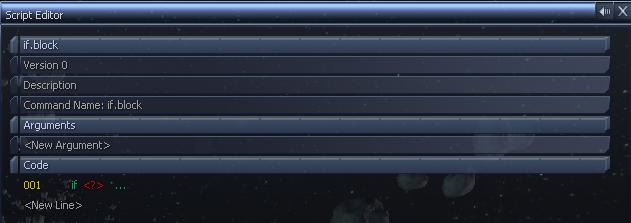You then need to fill in the expression, there are lots of commands you can use to do this, the simple ones can be used to check if a varible equals somes. The first varible will be the varible which you want to check, for this example we're going to use \$varible.Next, use the equals command, this is the "==" command. You use the "..." to add each command to the expression, you can keep adding commands.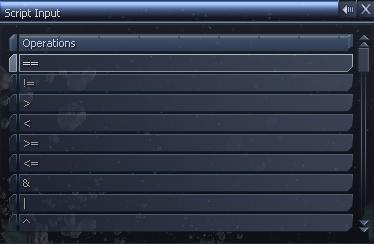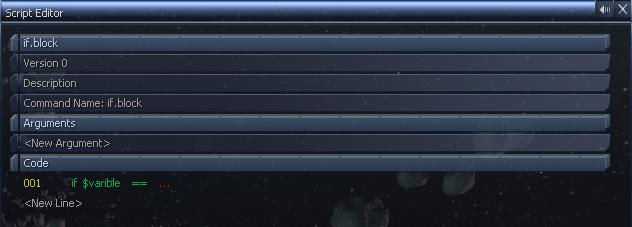Then final command is what to compare it too, this could be another varible to check if 2 varibles are the same, could also be a number or string. For this example, just goign to use the string "test".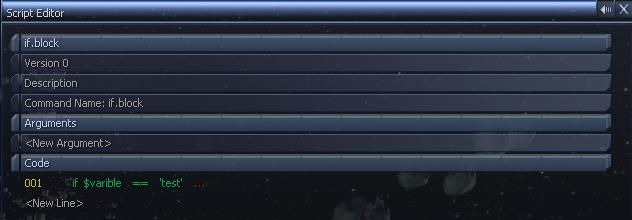Now, any commands added under this statement will only be used if \$varible equals the string "test". Otherwise they will be skipped. For this example we're just going to print a message to the logbook.Finally, you need to complete the block with the end statement, this is found in the General Commands menu, under the Flow Control sub menu.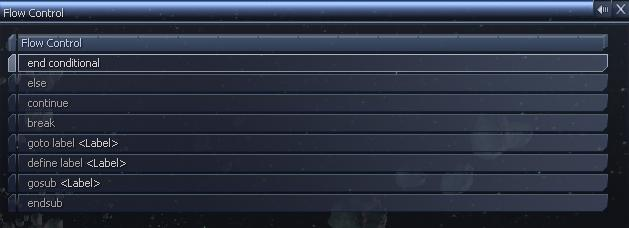This will finish off the If Block, notice that the commands between the If and End statements are indented slightly. You can have as many commands as you like between the block, and well as having nested if block, if blocks inside of other if blocks, etc.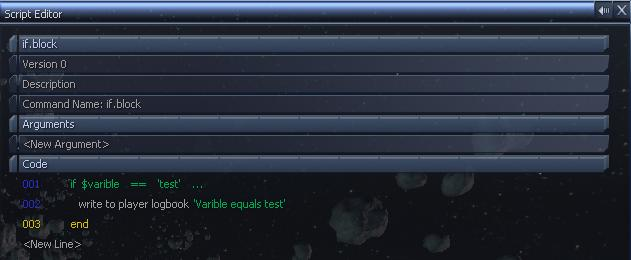## Skip If Statements

Skip if statements work like If Blocks, but you dont use it in a block, instead its used for single commands. And if the statement is true, it "skips" the next statement, which must be the line directly below.

The skip statement works he opposite to if statements, with if statement the commands in the blocks are only done if the statement is true, but with the skip if statement, the command below is done if the statement is false.

To get it the same as an if statement, you can use the command, skip if not, which turns a true statement into a false one.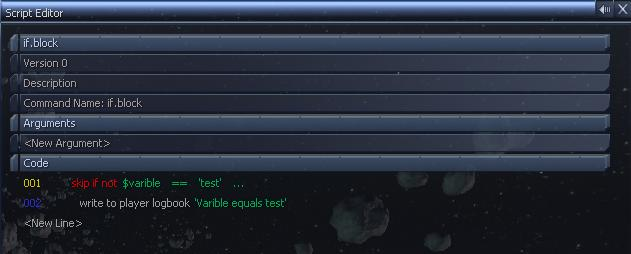## Else Statements

Else statements follow on from if statements, so if the statement was false, it will go into the else block. To add an else block, you put the "else" command inside the if block with the end command at the end of the whole block after the else command. Every command between else and end will be run if the original if statement is false.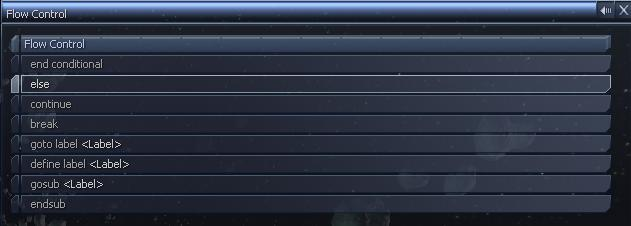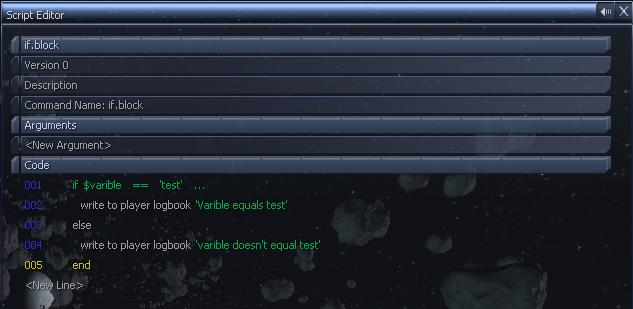You can only have 1 else statement in the if block and comes at the end of the block.

## Else/If Statement

Like with the else command, you can also have else if. So instead of always going into the else block, you can have another expression to check for. Heres an example but picking a random set of instructions.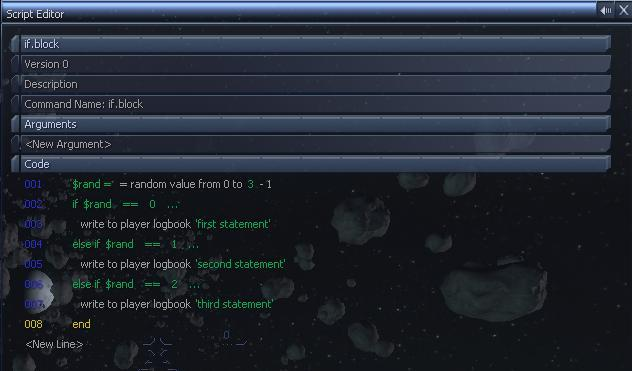You can also add the "else" command at the end, then all the commands in that block will be done if none of the previous statements are true.

Only the first true statement block will be done.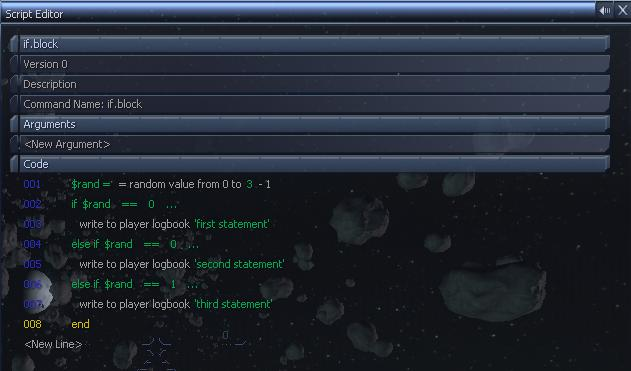This this example, if \$rand is 0 then the first and second statements are true. But only the first one will be done as it only does one set of statements in the whole block.

## While Block

A while block works in a simlar way to the if statements, you have the statement at the top with "end" at the bottom. But instead it will keep repeating the block while the statement is true. This can be used if you wish to run a command a certain amount of times. You create the statement in the same way as the if statement, but selecting the command "while" as the first one.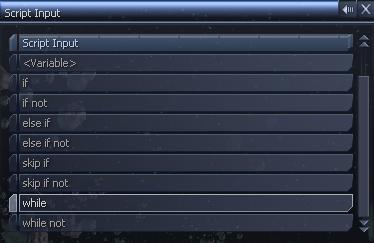In the example below, the statement block will run while the varible \$count is greater than 0, and each loop it decresed the value in \$count by one, ie counts down till 0.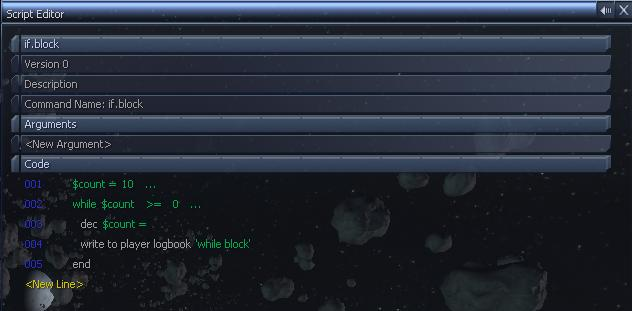the command "dec \$count =" means decrement the varible \$count, this only works if the varible is set to a number, so it will go from 10 to 9. So you will have 10 messages in your logbook after running it.

## Multiple Conditions

You can have conditions in the statement, ie you can test if 2 varibles equal something. Using the "AND" and "OR" commands between each statement. Each statement will be either true or false, if you use the "AND" statement, then both conditions must equal true for the commands to be run. For the "OR" statement, then either condition could equal true for the commands to be run. You can have as many conditions as you like each being seperated buy the AND or OR.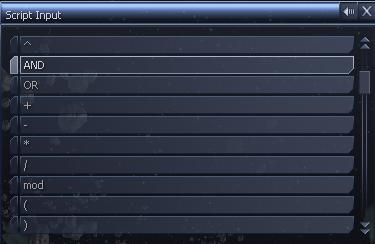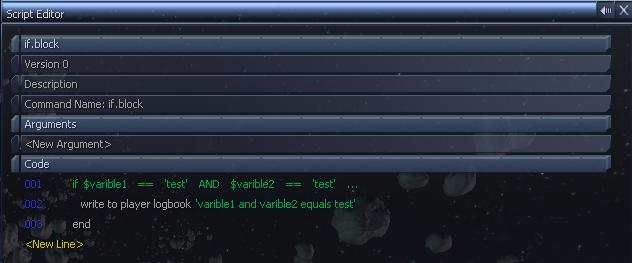In this, both \$varible1 and \$varible2 have to equal the string "test".

You can use brackets to change the prority of the statements as well as grouping them together.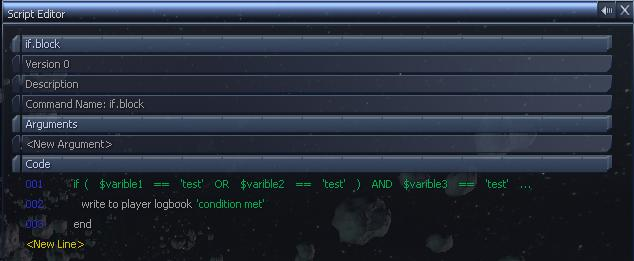In this example, the statements between the brackets will be checked first and put into one statement. So if you think of it as replacing each statement with ture or false, you get something like.

 if ( true or true ) and true if ( true ) and true true if ( true or false ) and true if ( true ) and true true if ( false or false ) and true if ( false ) and true false if ( false or true ) and false if ( true ) and false false

The first column replaces each statement with true or false, then the second removes the brackets. The third is if the whole statement is true or false.

You can use the "Home" and "End" keys to insert and remove commands in an expression. If you can add brackets after creating the whole expression, so if you highlight \$varible1 in the expression and press Home then it will insert a new command before it which you can set to the open bracket (.

`<< Prev (Using Command Slots)        Next (Using Arguments) >>`